# PHYS 1443 Section 001 Lecture 13 Wednesday June

• Slides: 20
Download presentation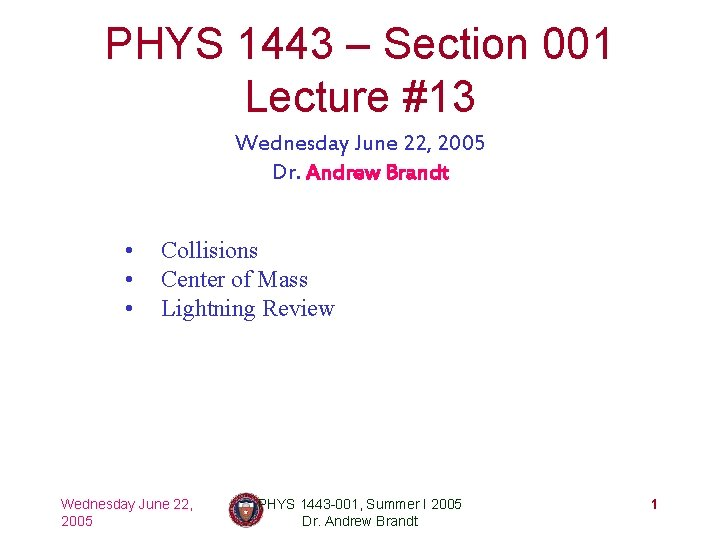PHYS 1443 – Section 001 Lecture #13 Wednesday June 22, 2005 Dr. Andrew Brandt • • • Collisions Center of Mass Lightning Review Wednesday June 22, 2005 PHYS 1443 -001, Summer I 2005 Dr. Andrew Brandt 1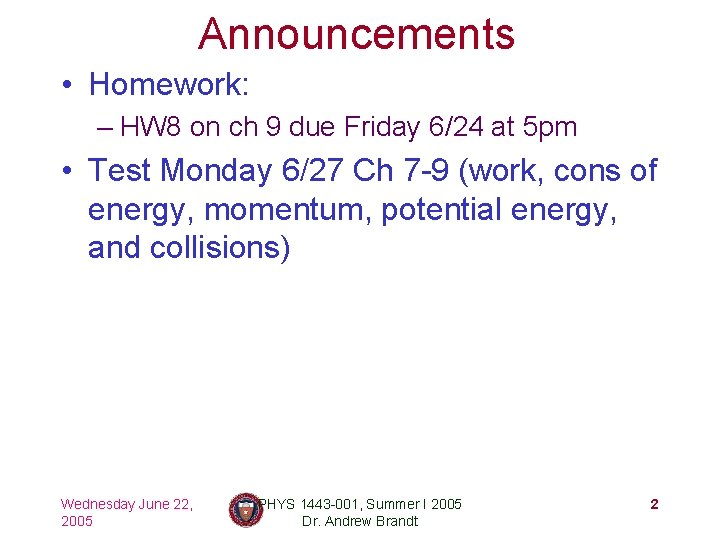Announcements • Homework: – HW 8 on ch 9 due Friday 6/24 at 5 pm • Test Monday 6/27 Ch 7 -9 (work, cons of energy, momentum, potential energy, and collisions) Wednesday June 22, 2005 PHYS 1443 -001, Summer I 2005 Dr. Andrew Brandt 2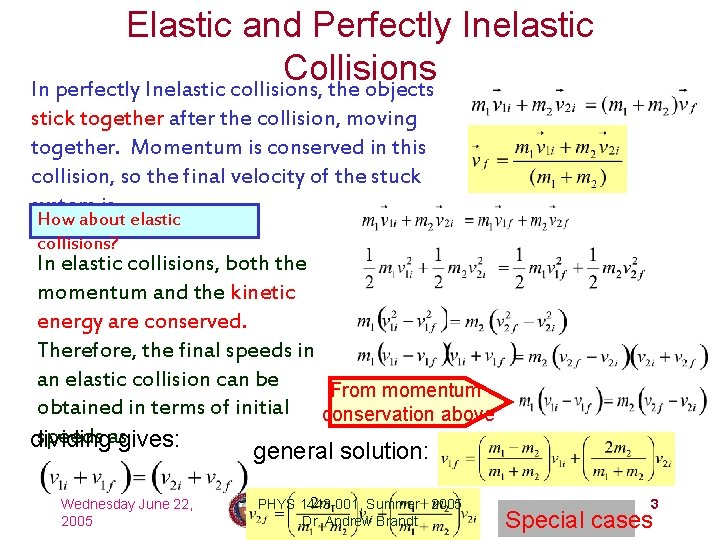Elastic and Perfectly Inelastic Collisions In perfectly Inelastic collisions, the objects stick together after the collision, moving together. Momentum is conserved in this collision, so the final velocity of the stuck system is How about elastic collisions? In elastic collisions, both the momentum and the kinetic energy are conserved. Therefore, the final speeds in an elastic collision can be From momentum obtained in terms of initial conservation above speeds asgives: dividing general solution: Wednesday June 22, 2005 PHYS 1443 -001, Summer I 2005 Dr. Andrew Brandt 3 Special casesExample for Collisions A car of mass 1800 kg stopped at a traffic light is rear-ended by a 900 kg car, and the two become entangled. If the lighter car was moving at 20. 0 m/s before the collision what is the velocity of the entangled cars after the collision? The momenta before and after the Before collision are collision m 2 20. 0 m/s m 1 After collision m 2 vf Since momentum of the system must be conserved m 1 What can we learn from these The cars are moving in the same direction as equations about the direction and the lighter car’s original direction to conserve magnitude of the velocity before momentum. and after the collision? Wednesday June 22, PHYS 1443 -001, Summer I 2005 The magnitude is inversely proportional to 4 the 2005 Dr. total Andrew Brandt mass.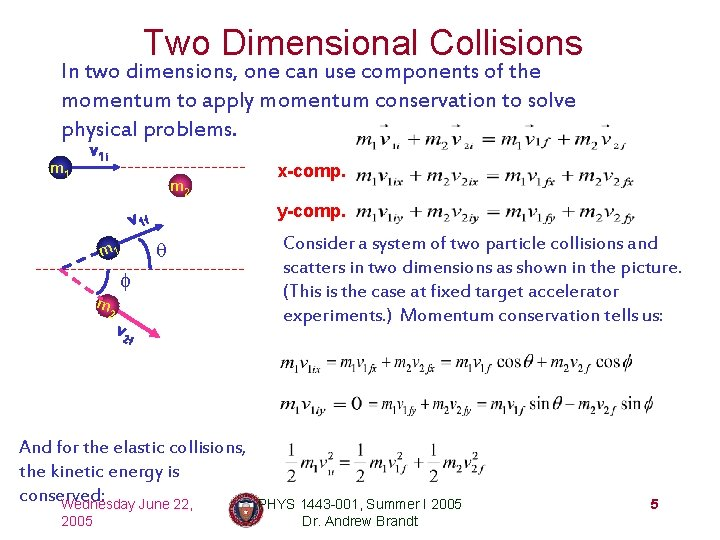Two Dimensional Collisions In two dimensions, one can use components of the momentum to apply momentum conservation to solve physical problems. m 1 v 1 i m 2 y-comp. v 1 f m 1 m 2 q f v 2 f And for the elastic collisions, the kinetic energy is conserved: Wednesday June 22, 2005 x-comp. Consider a system of two particle collisions and scatters in two dimensions as shown in the picture. (This is the case at fixed target accelerator experiments. ) Momentum conservation tells us: PHYS 1443 -001, Summer I 2005 Dr. Andrew Brandt 5Example for Two Dimensional Collisions Proton #1 with a speed 3. 50 x 10 m/s collides elastically with proton 5 #2 initially at rest. After the collision, proton #1 moves at an angle of 37 o to the horizontal axis and proton #2 deflects at an angle f to the same axis. Find the final speeds of the two protons and the scattering angle of proton #2, f. v 1 i Since both the particles are protons m 1 m. Using 1=m 2=m p. m 2 momentum conservation, one obtains x-comp. v 1 f m 1 m 2 q f v 2 f From kinetic energy conservation: Wednesday June 22, 2005 y-comp. Canceling mp and put in all known quantities, one obtains Solving Eqs. 1 -3 PHYS 1443 -001, Summer I 2005 Dr. Andrew Brandt Do the math at home 6Center of Mass We’ve been solving physical problems treating objects as point particles with mass, but in realistic situation each object has shape with the mass distributed throughout it. The center of mass of a system is the average position of the system’s mass and represents the motion of the system as if all the mass at that point. The total external force exerted on the What does above system of total mass M causes the center of statement tell you mass to move at an acceleration given by concerning forces as if all the mass of the system is being exerted on the concentrated at the center of mass. system? m 2 m 1 x 2 x. CM Wednesday June 22, 2005 Consider a massless rod with a ball attached at each end. of the center of mass of this The position system is the mass-averaged position of the system CM is closer to PHYS 1443 -001, Summer I 2005 Dr. Andrew Brandt the heavier object 7Motion of a Diver and the Center of Mass A diver performs a simple dive. The motion of the center of mass follows a parabola since it is a projectile motion. Wednesday June 22, 2005 A diver performs a complicated dive. The motion of the center of mass still follows the same parabola since it still is a projectile motion. The motion of the center of PHYS 1443 -001, Summer I 2005 8 mass of the diver is always Dr. Andrew BrandtExample 9 -12 Thee people of roughly equivalent mass M on a lightweight (air-filled) banana boat sit along the x axis at positions x 1=1. 0 m, x 2=5. 0 m, and x 3=6. 0 m. Find the position of the CM. Using the formula for CM Wednesday June 22, 2005 PHYS 1443 -001, Summer I 2005 Dr. Andrew Brandt 9Center of Mass of a Rigid Object The formula for CM can be expanded to rigid object or a system of many particles The position vector of the center of mass of a many particle system is A rigid body – an object with shape and size with mass ri spread throughout the body, r. CM ordinary objects – can be considered as a group of particles with mass mi densely spread throughout the given Wednesday June 22, PHYS 1443 -001, Summer I 2005 shape of the object 2005 Dr. Andrew Brandt Dmi 10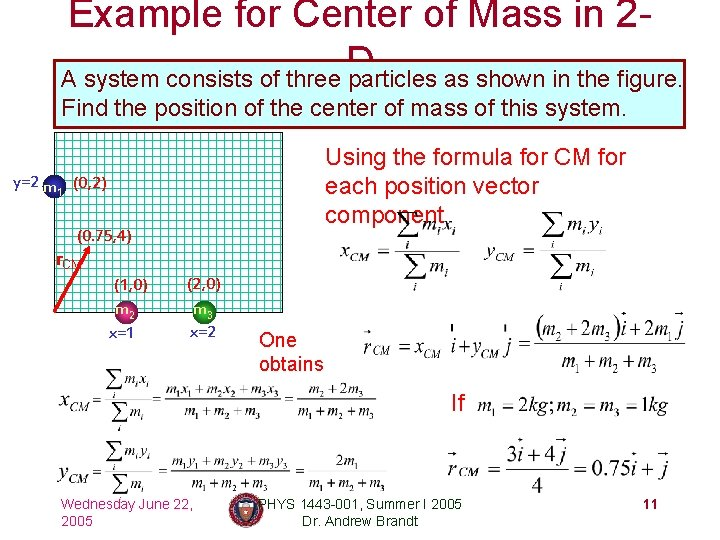Example for Center of Mass in 2 D A system consists of three particles as shown in the figure. Find the position of the center of mass of this system. Using the formula for CM for each position vector component y=2 m (0, 2) 1 (0. 75, 4) r. CM (1, 0) m 2 x=1 (2, 0) m 3 x=2 One obtains If Wednesday June 22, 2005 PHYS 1443 -001, Summer I 2005 Dr. Andrew Brandt 11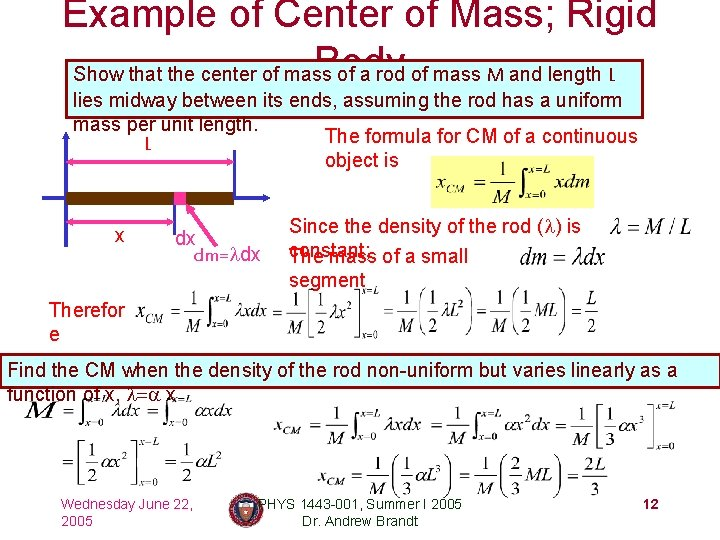Example of Center of Mass; Rigid Body Show that the center of mass of a rod of mass M and length L lies midway between its ends, assuming the rod has a uniform mass per unit length. The formula for CM of a continuous L object is x dx dm=ldx Since the density of the rod (l) is constant; The mass of a small segment Therefor e Find the CM when the density of the rod non-uniform but varies linearly as a function of x, l=a x Wednesday June 22, 2005 PHYS 1443 -001, Summer I 2005 Dr. Andrew Brandt 12Center of Mass and Center of Gravity CM The center of mass of any symmetric object lies on an axis of symmetry and on any plane of symmetry, if the object’s mass is evenly distributed Axis of throughout the body. One can use gravity to locate the CM. symmetr How do you think you can determine the CM of objects that are not symmetric? Center of Gravity Dmi 1. Hang the object by one point andydraw a vertical line following a plum-bob. 2. Hang the object by another point and do the same. 3. The pointobject where thebe two lines meetasis the Since a rigid can considered CM. of small masses, one can see collection the total gravitational force exerted on the object as The net effect of these small What does this gravitational forces is equivalent to a equation tell single force acting at a point (Center of Gravity) Wednesday Juneyou? 22, PHYS 1443 -001, Summer I 2005 with mass M. 13 Dmig The Co. G is the point in an object which can be treated as if all the gravitational 2005 Dr. Andrew Brandt force is acting on it!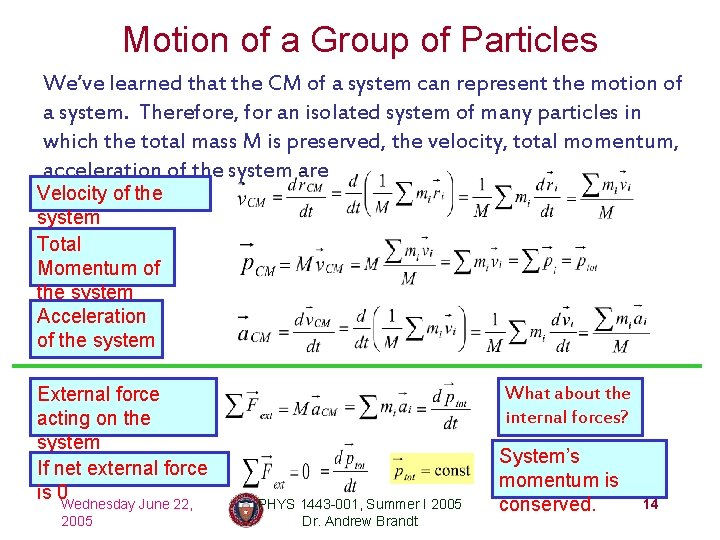Motion of a Group of Particles We’ve learned that the CM of a system can represent the motion of a system. Therefore, for an isolated system of many particles in which the total mass M is preserved, the velocity, total momentum, acceleration of the system are Velocity of the system Total Momentum of the system Acceleration of the system External force acting on the system If net external force is 0 Wednesday June 22, 2005 What about the internal forces? PHYS 1443 -001, Summer I 2005 Dr. Andrew Brandt System’s momentum is conserved. 14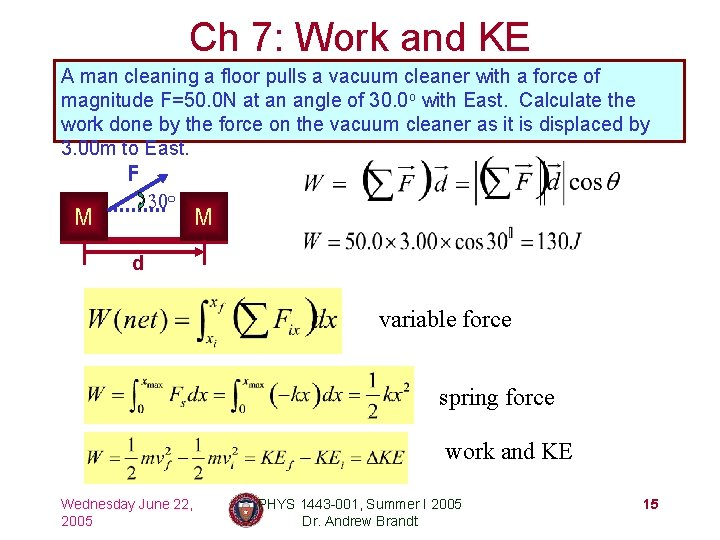Ch 7: Work and KE A man cleaning a floor pulls a vacuum cleaner with a force of magnitude F=50. 0 N at an angle of 30. 0 o with East. Calculate the work done by the force on the vacuum cleaner as it is displaced by 3. 00 m to East. F M 30 o M d variable force spring force work and KE Wednesday June 22, 2005 PHYS 1443 -001, Summer I 2005 Dr. Andrew Brandt 15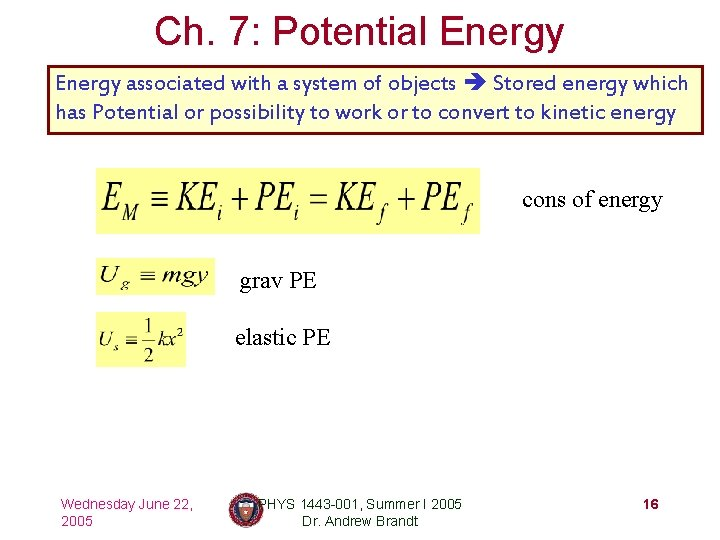Ch. 7: Potential Energy associated with a system of objects Stored energy which has Potential or possibility to work or to convert to kinetic energy cons of energy grav PE elastic PE Wednesday June 22, 2005 PHYS 1443 -001, Summer I 2005 Dr. Andrew Brandt 16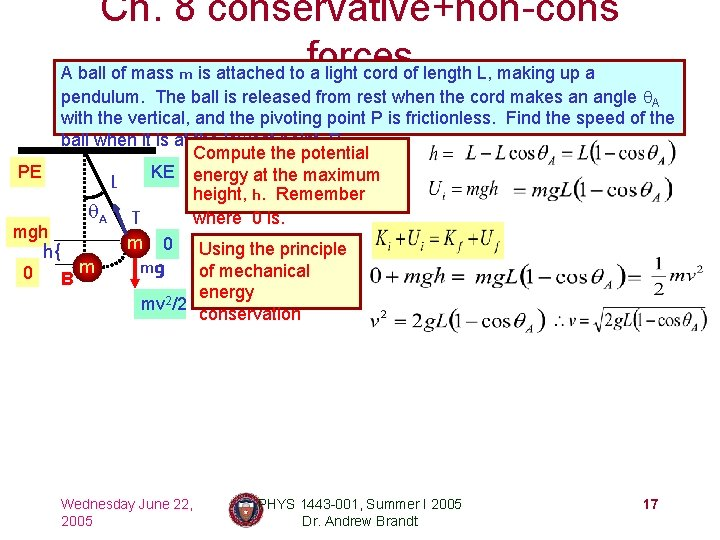Ch. 8 conservative+non-cons forces A ball of mass m is attached to a light cord of length L, making up a pendulum. The ball is released from rest when the cord makes an angle q. A with the vertical, and the pivoting point P is frictionless. Find the speed of the ball when it is at the lowest point, B. Compute the potential PE KE energy at the maximum L height, h. Remember q. A T where 0 is. mgh m 0 Using the principle h{ mg m of mechanical 0 B energy mv 2/2 conservation Wednesday June 22, 2005 PHYS 1443 -001, Summer I 2005 Dr. Andrew Brandt 17Work Done by non-conservative Forces Mechanical energy of a system is not conserved when any one of the forces in the system is a nonconservative force. Two kinds of non-conservative forces: Applied forces: Forces that are external to the system. These forces can take away or add energy to the system. So the mechanical energy of the system is no longer conserved. If you were to carry around a ball, the force you apply to the ball is external to the system of ball and the Earth. Therefore, you add kinetic energy to the ball. Earth system. Kinetic Friction: Internal non-conservative force that causes irreversible transformation of energy. The friction force causes the kinetic and potential energy to transfer to internal energy Wednesday June 22, 2005 PHYS 1443 -001, Summer I 2005 Dr. Andrew Brandt 18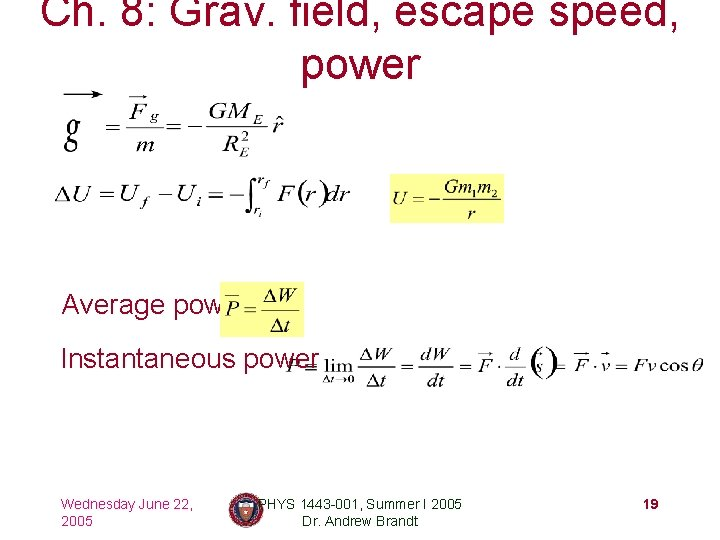Ch. 8: Grav. field, escape speed, power Average power Instantaneous power Wednesday June 22, 2005 PHYS 1443 -001, Summer I 2005 Dr. Andrew Brandt 19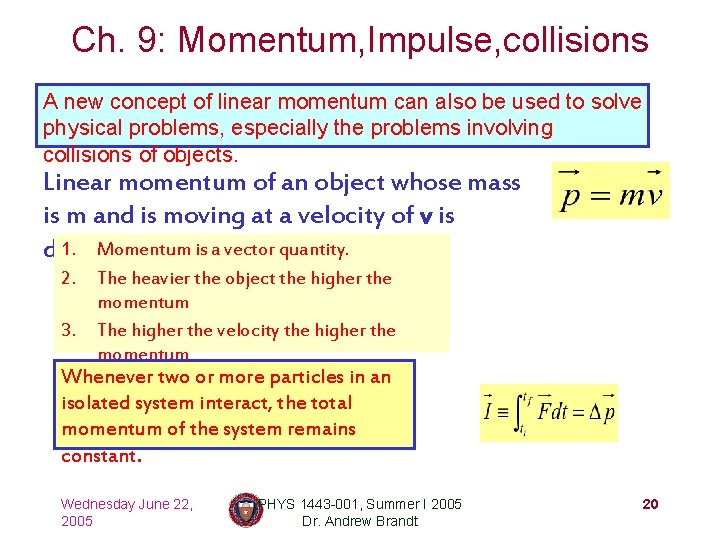Ch. 9: Momentum, Impulse, collisions A new concept of linear momentum can also be used to solve physical problems, especially the problems involving collisions of objects. Linear momentum of an object whose mass is m and is moving at a velocity of v is 1. Momentum defined as is a vector quantity. 2. The heavier the object the higher the momentum 3. The higher the velocity the higher the momentum Whenever or more particles in an 4. Its unit istwo kg. m/s isolated system interact, the total momentum of the system remains constant. Wednesday June 22, 2005 PHYS 1443 -001, Summer I 2005 Dr. Andrew Brandt 20